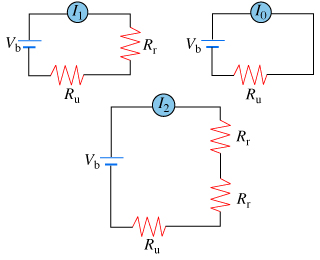# Problem: An ammeter is connected in series to a battery of voltage Vb and a resistor of unknown resistance Ru (Figure 1) . The ammeter reads a current Io. Next, a resistor of unknown resistance Rr is connected in series to the ammeter, and the ammeter's reading drops to I1. Finally, a second resistor, also of resistance Rr, is connected in series as well. Now the ammeter reads I2.If I1/I0=4/5, find I2/I0.Express the ratio I2/I0 numerically.I2/I0 =

###### FREE Expert Solution

Ohm's law:

$\overline{){\mathbf{I}}{\mathbf{=}}\frac{\mathbf{V}}{\mathbf{R}}}$

The current flowing in each circuit is expressed as:

I0 = Vb/RU

I1 = Vb/(R+ RU)

91% (252 ratings)###### Problem Details

An ammeter is connected in series to a battery of voltage Vb and a resistor of unknown resistance Ru (Figure 1) . The ammeter reads a current Io. Next, a resistor of unknown resistance Rr is connected in series to the ammeter, and the ammeter's reading drops to I1. Finally, a second resistor, also of resistance Rr, is connected in series as well. Now the ammeter reads I2.

If I1/I0=4/5, find I2/I0.

Express the ratio I2/I0 numerically.

I2/I0 =